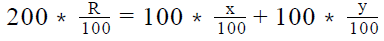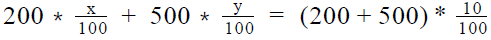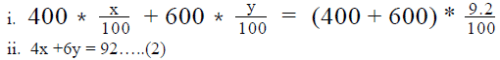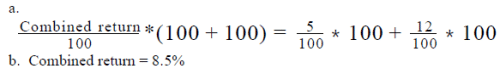A \$200 investment at x percent per annum and ...
A \$200 investment at x percent per annum and a \$500 investment at y percent per annum have a combined yearly return of 10 percent of the total of the two investments. If \$400 is invested at x percent and \$600 is invested at y percent per annum to give a combined yearly return of 9.2 percent of the total of the two investments, what will be the combined percentage yearly return of the total investment if \$100 each is invested at x percent per annum and y percent per annum respectively?
• a)
5%
• b)
7.5%
• c)
8.5%
• d)
10%
• e)
17%
A \$200 investment at x percent per annum and a \$500 investment at y pe...
Given:
• Case-I
• Principal , P = \$200
• Rate of interest = x% p.a.
• Principal, P = \$500
• Rate of Interest = y% p.a.
• Combined yearly return = 10%
• Case-II
• Principal, P = \$400
• Rate of interest = x% p.a.
• Principal, P = \$600
• Rate of Interest = y% p.a.
• Combined yearly return = 9.2%
To Find: Combined yearly return if \$100 each is invested at x% p.a. and y% p.a.?
• Let the combined yearly return be R%
• So, Return on \$200 invested at R% p.a. = Return on \$100 at x% p.a. + Return on \$100 at y% p.a.
•Approach:
1. For finding the value of R, we need to find the value of x and y.
2. We are given two cases in which the principals are invested at x% and y% per annum and we are also given the combined yearly return for both the cases
• The combined yearly return is equal to the sum of the returns of the individual investments
• Writing the combined yearly return equations for each of the case will give us an equation in x and y
3. We will solve both the equations to get the value of x and y, which will be used to calculate the yearly return on \$100 each invested at x% and y% p.a.
Working out:
1. Case-I
• As the combined yearly return is equal to the sum of the returns of individual investments, we can write the following equation
•• 2x +5y = 70……………..(1)
2. Case-II
• As the combined yearly return is equal to the sum of the returns of individual investments, we can write the following equation3. Solving (1) and (2), we have y = 12 and x = 5
4. Hence, combined yearly return on investment of \$100 each at x% and y% can be calculated asA \$200 investment at x percent per annum and a \$500 investment at y pe...
Given:
• Case-I
• Principal , P = \$200
• Rate of interest = x% p.a.
• Principal, P = \$500
• Rate of Interest = y% p.a.
• Combined yearly return = 10%
• Case-II
• Principal, P = \$400
• Rate of interest = x% p.a.
• Principal, P = \$600
• Rate of Interest = y% p.a.
• Combined yearly return = 9.2%
To Find: Combined yearly return if \$100 each is invested at x% p.a. and y% p.a.?
• Let the combined yearly return be R%
• So, Return on \$200 invested at R% p.a. = Return on \$100 at x% p.a. + Return on \$100 at y% p.a.
•Approach:
1. For finding the value of R, we need to find the value of x and y.
2. We are given two cases in which the principals are invested at x% and y% per annum and we are also given the combined yearly return for both the cases
• The combined yearly return is equal to the sum of the returns of the individual investments
• Writing the combined yearly return equations for each of the case will give us an equation in x and y
3. We will solve both the equations to get the value of x and y, which will be used to calculate the yearly return on \$100 each invested at x% and y% p.a.
Working out:
1. Case-I
• As the combined yearly return is equal to the sum of the returns of individual investments, we can write the following equation
•• 2x +5y = 70……………..(1)
2. Case-II
• As the combined yearly return is equal to the sum of the returns of individual investments, we can write the following equation3. Solving (1) and (2), we have y = 12 and x = 5
4. Hence, combined yearly return on investment of \$100 each at x% and y% can be calculated asAttention GMAT Students!
To make sure you are not studying endlessly, EduRev has designed GMAT study material, with Structured Courses, Videos, & Test Series. Plus get personalized analysis, doubt solving and improvement plans to achieve a great score in GMAT.
 1 Crore+ students have signed up on EduRev. Have you?
1 Crore+ students have signed up on EduRev. Have you?

### Learn this topic in detailTest: Savings And Interest- 2 19 Ques | 40 MinsMore from Related Course Quantitative for GMATView courses related to this question

### Quick links for GMAT exam850+
Video Lectures2500+
Revision Notes600+
Online Tests10,000+
Doubts Solved
A \$200 investment at x percent per annum and a \$500 investment at y percent per annum have a combined yearly return of 10 percent of the total of the two investments. If \$400 is invested at x percent and \$600 is invested at y percent per annum to give a combined yearly return of 9.2 percent of the total of the two investments, what will be the combined percentage yearly return of the total investment if \$100 each is invested at x percent per annum and y percent per annum respectively?a)5%b)7.5%c)8.5%d)10%e)17%Correct answer is option 'C'. Can you explain this answer?
Question Description
A \$200 investment at x percent per annum and a \$500 investment at y percent per annum have a combined yearly return of 10 percent of the total of the two investments. If \$400 is invested at x percent and \$600 is invested at y percent per annum to give a combined yearly return of 9.2 percent of the total of the two investments, what will be the combined percentage yearly return of the total investment if \$100 each is invested at x percent per annum and y percent per annum respectively?a)5%b)7.5%c)8.5%d)10%e)17%Correct answer is option 'C'. Can you explain this answer? for GMAT 2023 is part of GMAT preparation. The Question and answers have been prepared according to the GMAT exam syllabus. Information about A \$200 investment at x percent per annum and a \$500 investment at y percent per annum have a combined yearly return of 10 percent of the total of the two investments. If \$400 is invested at x percent and \$600 is invested at y percent per annum to give a combined yearly return of 9.2 percent of the total of the two investments, what will be the combined percentage yearly return of the total investment if \$100 each is invested at x percent per annum and y percent per annum respectively?a)5%b)7.5%c)8.5%d)10%e)17%Correct answer is option 'C'. Can you explain this answer? covers all topics & solutions for GMAT 2023 Exam. Find important definitions, questions, meanings, examples, exercises and tests below for A \$200 investment at x percent per annum and a \$500 investment at y percent per annum have a combined yearly return of 10 percent of the total of the two investments. If \$400 is invested at x percent and \$600 is invested at y percent per annum to give a combined yearly return of 9.2 percent of the total of the two investments, what will be the combined percentage yearly return of the total investment if \$100 each is invested at x percent per annum and y percent per annum respectively?a)5%b)7.5%c)8.5%d)10%e)17%Correct answer is option 'C'. Can you explain this answer?.
Solutions for A \$200 investment at x percent per annum and a \$500 investment at y percent per annum have a combined yearly return of 10 percent of the total of the two investments. If \$400 is invested at x percent and \$600 is invested at y percent per annum to give a combined yearly return of 9.2 percent of the total of the two investments, what will be the combined percentage yearly return of the total investment if \$100 each is invested at x percent per annum and y percent per annum respectively?a)5%b)7.5%c)8.5%d)10%e)17%Correct answer is option 'C'. Can you explain this answer? in English & in Hindi are available as part of our courses for GMAT. Download more important topics, notes, lectures and mock test series for GMAT Exam by signing up for free.
Here you can find the meaning of A \$200 investment at x percent per annum and a \$500 investment at y percent per annum have a combined yearly return of 10 percent of the total of the two investments. If \$400 is invested at x percent and \$600 is invested at y percent per annum to give a combined yearly return of 9.2 percent of the total of the two investments, what will be the combined percentage yearly return of the total investment if \$100 each is invested at x percent per annum and y percent per annum respectively?a)5%b)7.5%c)8.5%d)10%e)17%Correct answer is option 'C'. Can you explain this answer? defined & explained in the simplest way possible. Besides giving the explanation of A \$200 investment at x percent per annum and a \$500 investment at y percent per annum have a combined yearly return of 10 percent of the total of the two investments. If \$400 is invested at x percent and \$600 is invested at y percent per annum to give a combined yearly return of 9.2 percent of the total of the two investments, what will be the combined percentage yearly return of the total investment if \$100 each is invested at x percent per annum and y percent per annum respectively?a)5%b)7.5%c)8.5%d)10%e)17%Correct answer is option 'C'. Can you explain this answer?, a detailed solution for A \$200 investment at x percent per annum and a \$500 investment at y percent per annum have a combined yearly return of 10 percent of the total of the two investments. If \$400 is invested at x percent and \$600 is invested at y percent per annum to give a combined yearly return of 9.2 percent of the total of the two investments, what will be the combined percentage yearly return of the total investment if \$100 each is invested at x percent per annum and y percent per annum respectively?a)5%b)7.5%c)8.5%d)10%e)17%Correct answer is option 'C'. Can you explain this answer? has been provided alongside types of A \$200 investment at x percent per annum and a \$500 investment at y percent per annum have a combined yearly return of 10 percent of the total of the two investments. If \$400 is invested at x percent and \$600 is invested at y percent per annum to give a combined yearly return of 9.2 percent of the total of the two investments, what will be the combined percentage yearly return of the total investment if \$100 each is invested at x percent per annum and y percent per annum respectively?a)5%b)7.5%c)8.5%d)10%e)17%Correct answer is option 'C'. Can you explain this answer? theory, EduRev gives you an ample number of questions to practice A \$200 investment at x percent per annum and a \$500 investment at y percent per annum have a combined yearly return of 10 percent of the total of the two investments. If \$400 is invested at x percent and \$600 is invested at y percent per annum to give a combined yearly return of 9.2 percent of the total of the two investments, what will be the combined percentage yearly return of the total investment if \$100 each is invested at x percent per annum and y percent per annum respectively?a)5%b)7.5%c)8.5%d)10%e)17%Correct answer is option 'C'. Can you explain this answer? tests, examples and also practice GMAT tests.(Scan QR code)